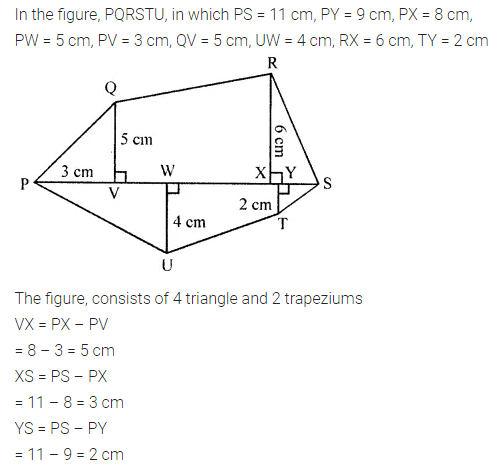# ML Aggarwal Class 8 Solutions for ICSE Maths Chapter 18 Mensuration Ex 18.2

## ML Aggarwal Class 8 Solutions for ICSE Maths Chapter 18 Mensuration Ex 18.2

Question 1.
Each side of a rhombus is 13 cm and one diagonal is 10 cm. Find
(i) the length of its other diagonal
(ii) the area of the rhombus
Solution: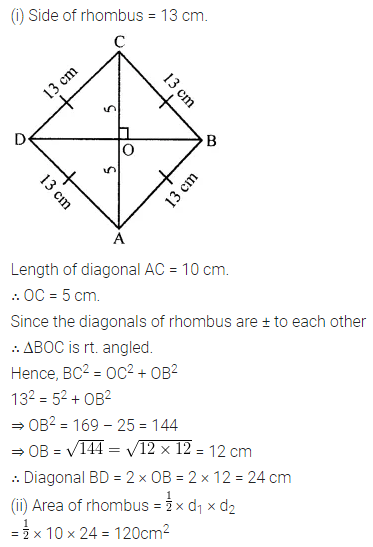Question 2.
The cross-section ABCD of a swimming pool is a trapezium. Its width AB = 14 m, depth at the shallow end is 1-5 m and at the deep end is 8 m. Find the area of the cross-section.Solution: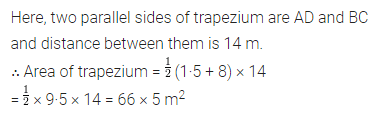Question 3.
The area of a trapezium is 360 m2, the distance between two parallel sides is 20 m and one of the parallel side is 25 m. Find the other parallel side.
Solution:Question 4.
Find the area of a rhombus whose side is 6.5 cm and altitude is 5 cm. If one of its diagonal is 13 cm long, find the length of other diagonal.
Solution: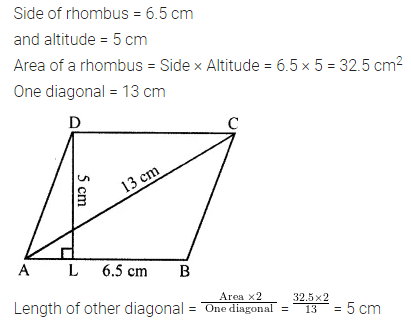Question 5.
From the given diagram, calculate
(i) the area of trapezium ACDE
(ii) the area of parallelogram ABDE
(iii) the area of triangle BCD.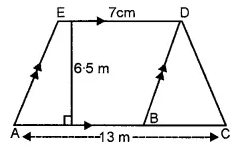Solution:Question 6.
The area of a rhombus is equal to the area of a triangle whose base and the corresponding altitude are 24.8 cm and 16.5 cm respectively. If one of the diagonals of the rhombus is 22 cm, find the length of the other diagonal.
Solution:Question 7.
The perimeter of a trapezium is 52 cm. If its non-parallel sides are 10 cm each and its altitude is 8 cm, find the area of the trapezium.
Solution: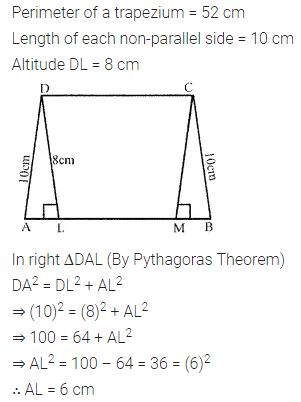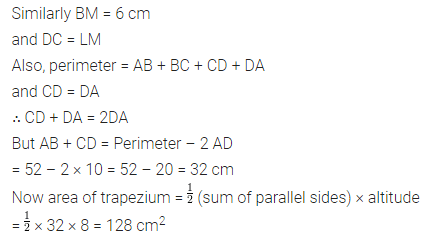Question 8.
The area of a trapezium is 540 cm2. If the ratio of parallel sides is 7 : 5 and the distance between them is 18 cm, find the lengths of parallel sides.
Solution: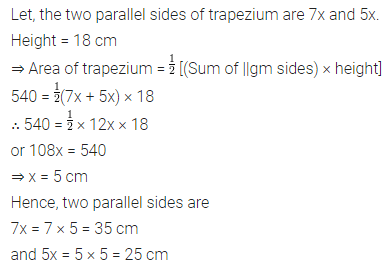Question 9.
Calculate the area enclosed by the given shapes. All measurements are in cm.Solution: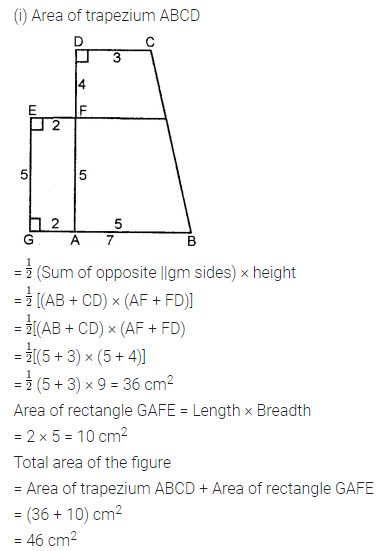Question 10.
(ii) the area of trapezium ABCD
(iii) the area of triangle BCD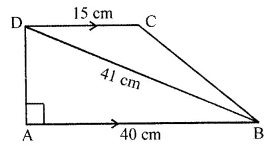Solution: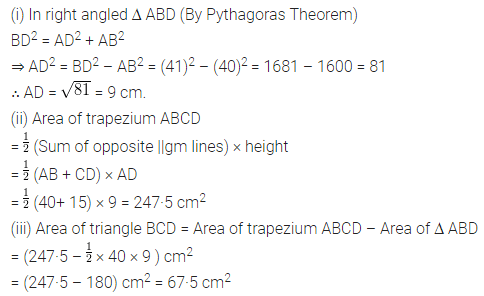Question 11.
Diagram of the adjacent picture frame has outer dimensions = 28 cm × 32 cm and inner dimensions 20 cm × 24 cm. Find the area of each section of the frame, if the width of each section is same.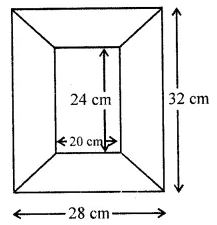Solution:Question 12.
In the given quadrilateral ABCD, ∠BAD = 90° and ∠BDC = 90°. All measurements are in centimetres. Find the area of the quadrilateral ABCD.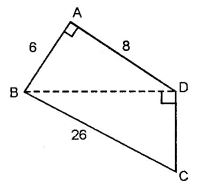Solution:Question 13.
Top surface of a raised platform is in the shape of a regular octagon as shown in the given figure. Find the area of the octagonal surface.Solution: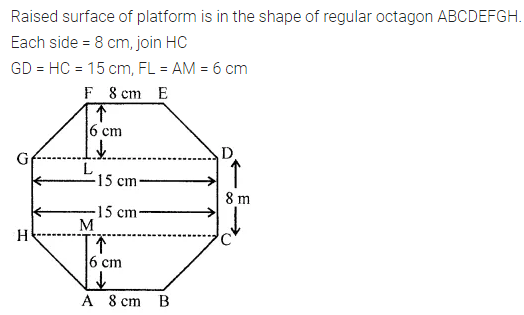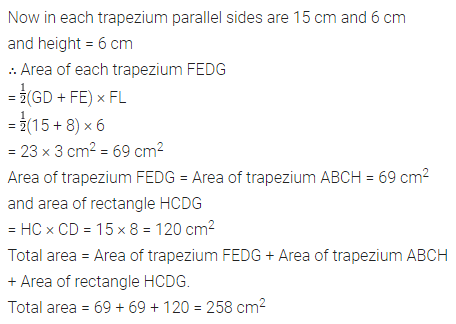Question 14.
There is a pentagonal shaped park as shown in the following figure:
For finding its area Jaspreet and Rahul divided it in two different ways.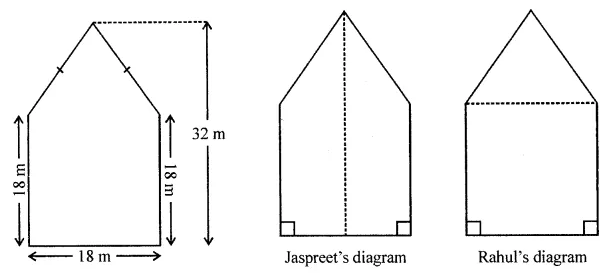Find the area of this park using both ways. Can you suggest some other way of finding its area?
Solution: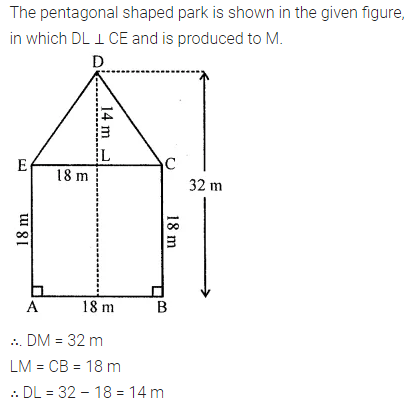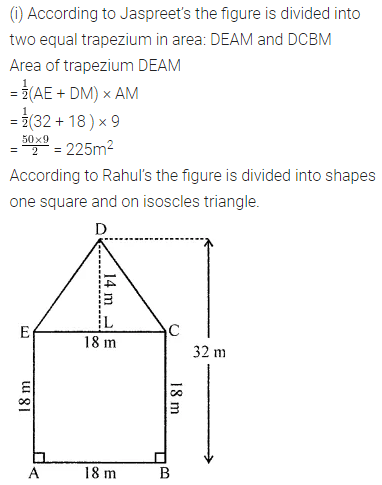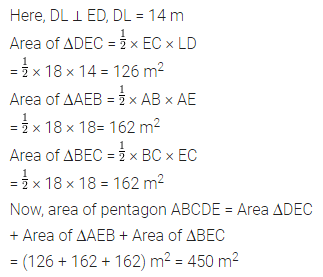Question 15.
In the diagram, ABCD is a rectangle of size 18 cm by 10 cm. In ∆ BEC, ∠E = 90° and EC = 8 cm. Find the area enclosed by the pentagon ABECD.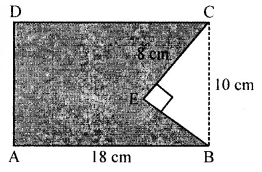Solution:Question 16.
Polygon ABCDE is divided into parts as shown in the given figure. Find its area if AD = 8 cm, AH = 6 cm, AG = 4 cm, AF = 3 cm and perpendiculars BF = 2 cm, CH = 3 cm, EG = 2.5 cm.Solution: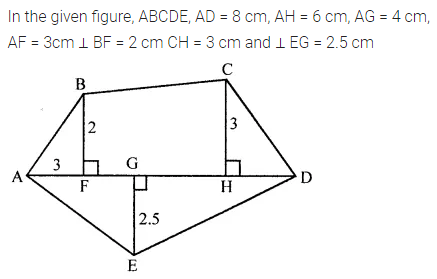Question 17.
Find the area of polygon PQRSTU shown in 1 the given figure, if PS = 11 cm, PY = 9 cm, PX = 8 cm, PW = 5 cm, PV = 3 cm, QV = 5 cm, UW = 4 cm, RX = 6 cm, TY = 2 cm.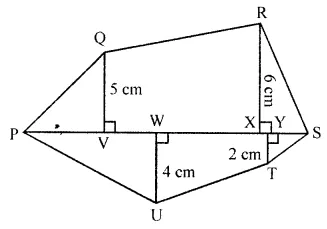Solution: